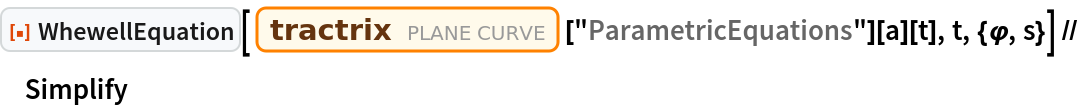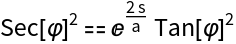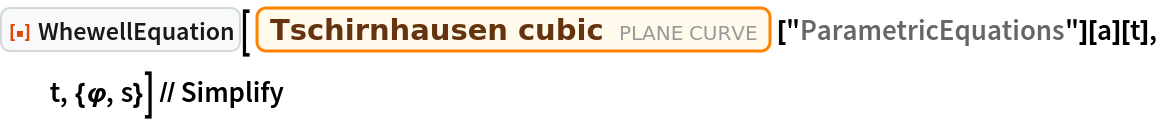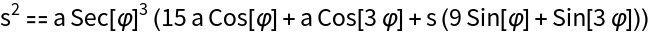Function Repository Resource:

# WhewellEquation

Compute the Whewell equation for a plane curve

Contributed by: Jan Mangaldan
 ResourceFunction["WhewellEquation"][c,t,{φ,s}] computes the Whewell equation for tangential angle φ and arc length s of a plane curve c parametrized by t.

## Details

The Whewell equation is a natural equation that expresses a curve in terms of its arc length s and tangential angle φ.

## Examples

### Basic Examples (2)

Whewell equation for the tractrix:

 In:=Out=Whewell equation for the Tschirnhausen cubic:

 In:=Out=## Version History

• 1.0.0 – 09 March 2021